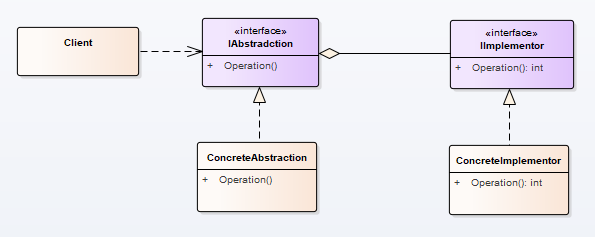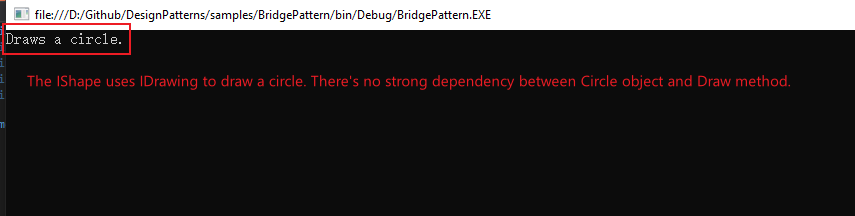桥接模式 (Bridge Pattern)-结构型模式第五篇

UML：实例：

using System;
using System.Collections.Generic;
using System.Linq;
using System.Text;

namespace BridgePattern
{

/// <summary>
/// This is abstraction, represents shape.
/// </summary>
public interface IShape
{
/// <summary>
/// The shape has a property called Drawing, which represents implemention of drawing behavior.
/// </summary>
IDrawing Drawing { get; set; }
/// <summary>
/// The shape has a method called Draw. If you don't adopt Bridge pattern, in general, you should implement this method to draw.
/// </summary>
/// <returns></returns>
string Draw();
}

/// <summary>
/// This is a concrete abstraction. represents circle shape.
/// </summary>
public class CircleShape : IShape
{
public CircleShape(IDrawing drawing)
{
Drawing = drawing;
}
public IDrawing Drawing { get; set; }
/// <summary>
/// The method Draw uses IDrawing to bridge. You will find that you don't need to implement concrete darwing behavior, you just forward the request to IDrawing.
/// </summary>
/// <returns></returns>
public string Draw()
{
return Drawing.Draw();
}
}

/// <summary>
/// This is abstraction of implementation, represents abstract implementation, it has ability to independent with IShape.
/// </summary>

public interface IDrawing
{
string Draw();
}

/// <summary>
/// This is concrete implementation, derived from IDrawing.
/// </summary>
public class CircleDrawing : IDrawing
{
public string Draw()
{
return "Draws a circle.";
}
}

class Program
{
static void Main(string[] args)
{
IDrawing drawing = new CircleDrawing(); //Get a circle drawing which will be used later.
IShape shape = new CircleShape(drawing);// Get a circle shape which uses drawing object to draw.
Console.WriteLine(shape.Draw());//Ok, shape draws.